edHelper subscribers - Create a new printable

Sample edHelper.com - Visual Perceptual Skills Worksheet

 Name _____________________________Date ___________________ (Answer ID # 0887688)
Pick the choice that is a wrong statement.
1.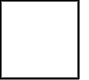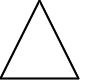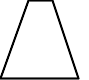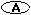the trapezoid is to the right of the triangle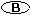the trapezoid is third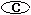the square is to the right of the trapezoid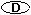the triangle is between the trapezoid and the square
2.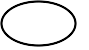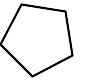the square is to the left of the pentagonthe oval is to the left of the pentagonthe pentagon is to the left of the squarethe pentagon is second
3.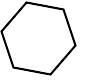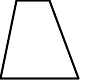the trapezoid is thirdthe square is to the right of the hexagonthe hexagon is to the left of the trapezoidthe hexagon is to the right of the square
4.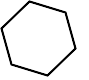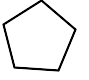the pentagon is to the left of the trapezoidthe hexagon is to the left of the pentagonthe trapezoid is firstthe trapezoid is to the left of the pentagon
5.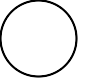the circle is to the right of the pentagonthe pentagon is between the circle and the trianglethe pentagon is between the triangle and the circlethe circle is first
6.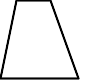the oval is to the right of the trapezoidthe trapezoid is between the circle and the ovalthe circle is to the left of the ovalthe oval is third